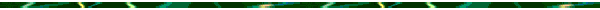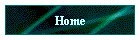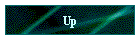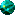If you need Tutoring in Math or Computer Science,
. Thanks!

Formula Sheets Chapters 4 - 16

This page has links to the formula sheets for Chapters 4 - 16.  In order to do your best on each test, benchmark, and semester exam you should know the formulas, what each symbol in the formula means, when to apply them, how to apply them, and practice using them to successfully solve problems.

Refer the "Knowledge Iceberg" as you memorize these formulas.

Refer to the Lecture Notes to see the formulas being applied to solve problems.Ch 3.pdfCh 4.pdfCh 7.pdfCh 8.pdfCh 9.pdfCh 10.pdfCh 11.pdfCh 12.pdfCh 15.pdfCh 16.pdf